# Tags: IF

In the value area section of a normal Pivot Table one can only show the result of aggregation functions such as SUM(), COUNT(), AVERAGE() etc.  Even if one drags a text field to the value area section of a Pivot Table, one cannot show those text fields because they automatically get counted.

Consider the following dataset.  The important columns to consider here are COD (Column C), Level (Column E) and Date (column G).For a COD, there can be a number of rows (COD 31512268 has 3 rows).  For this COD, there is just one level (E) for the same date/time.It is also possible that for a particular COD, there can be different Levels (COD 31512259 has 4 rows).  For this COD, there are 2 levels (E and D) for the same data/time.To further complicate the issue, there can be some cases where for the same date/time, a COD may have different levels.  COD 11058698 has 2 different levels (K and M) for the same date/time.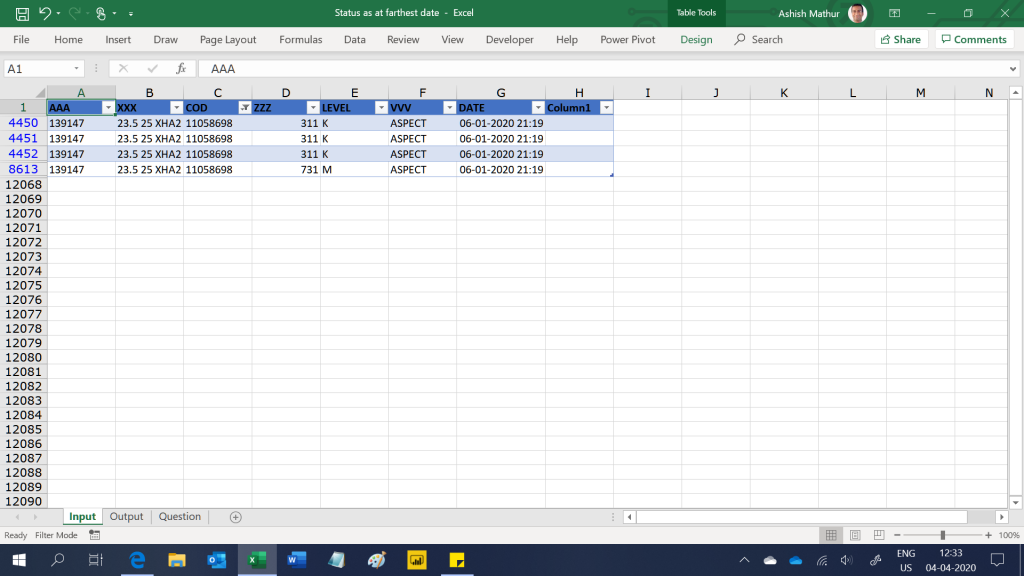The expected result is to show a Pivot Table with COD's in the row labels and the Level(s) as on the farthest date/time of each COD.  If a particular COD has 2 levels as on the farthest date/time, then they should be shown in the value area section of the Pivot Table (separated by commas).  So the expected result should look like this.  Notice that COD 11058698 has 2 levels as on the farthest date/time (K and M) and COD 11058700 has 3 levels as on the farthest date/time (Blank, M and 1M).I have solved this question in MS Excel and PowerBI Desktop with the help of the DAX formulas.  You may download my Excel solution workbook from here and PowerBI Desktop file from here.

Assume a simple 3 column dataset as shown below - the date of each task and the status of that task.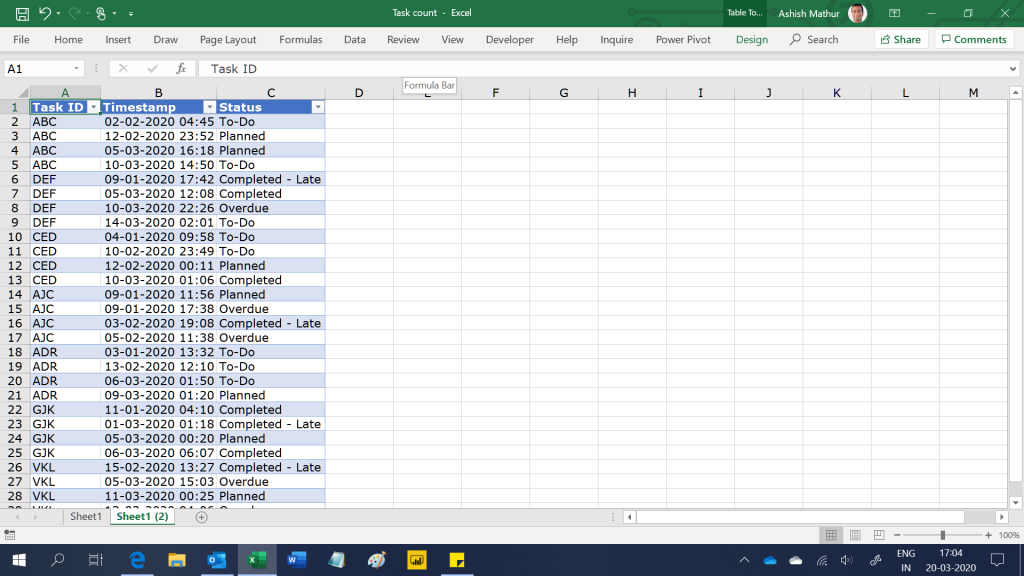The objective is to get the status wise count of tasks by the last time stamp.  So for the Status "To-do", the count should be 2 - Task ABC and DEF.  Only these two tasks on their last time stamp have the status as "To-do".  Tasks CED and ADR should not be counted because their last time stamp had a status other than "To-do".  So the final expected result in MS Excel is: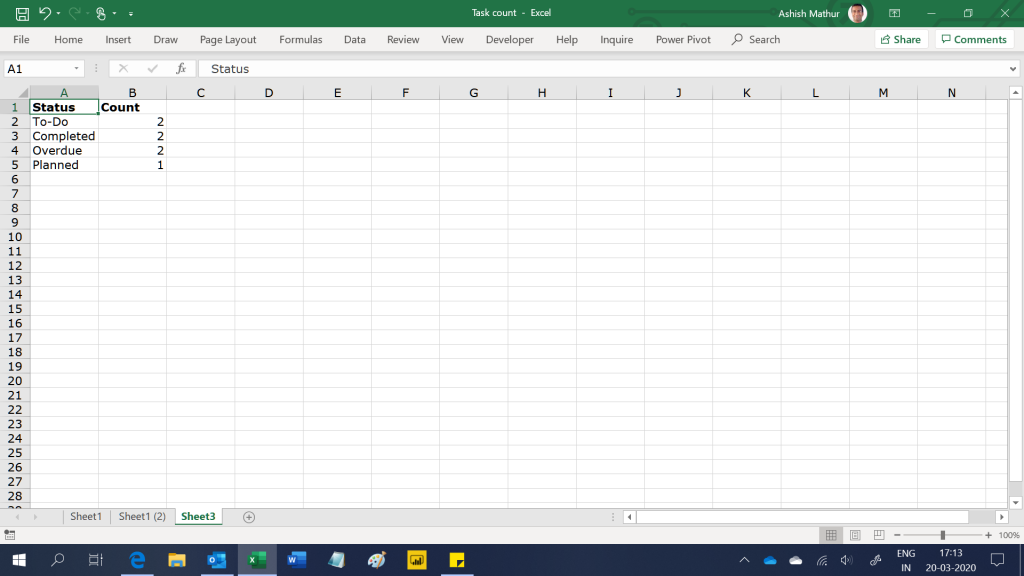Since the original data is being fetched from an external data source, no additional tables or columns can be created from/in the source data table.

The final result in PBI Desktop is thisYou may download my PowerPivot solution workbook from here and PBI Desktop solution file from here.

This post is an extension to the one I posted here - Segment towns according to volume contribution and market share. Here's a simple dataset of Shampoo sales in the state of Rajasthan, India.For a chosen segment, one may want to segment the 4 towns based on the following conditions:Based on the two screenshots shared above, the desired result is shown in the screenshot below:The difference between this solution at the previous one (the link of which I have shared above) is that in this one we want to drag the Classification (range E16:E17) to either the row/column/report filter section of the Pivot Table use it as a slicer.  The current limitation with measures that one writes in PowerPivot's is that measures cannot be used in either row/column/report filter section or as a slicer of/in a Pivot Table.  So in the previous solution, I had written a measure to return the result as Headroom, Stronghold, Emerging or small in only the value area section of the Pivot Table.  One could not drag that measure into the row labels of a Pivot Table.  In this solution, one can drag the Town classification to the row/column/report filter section or even to the slicer (see images below)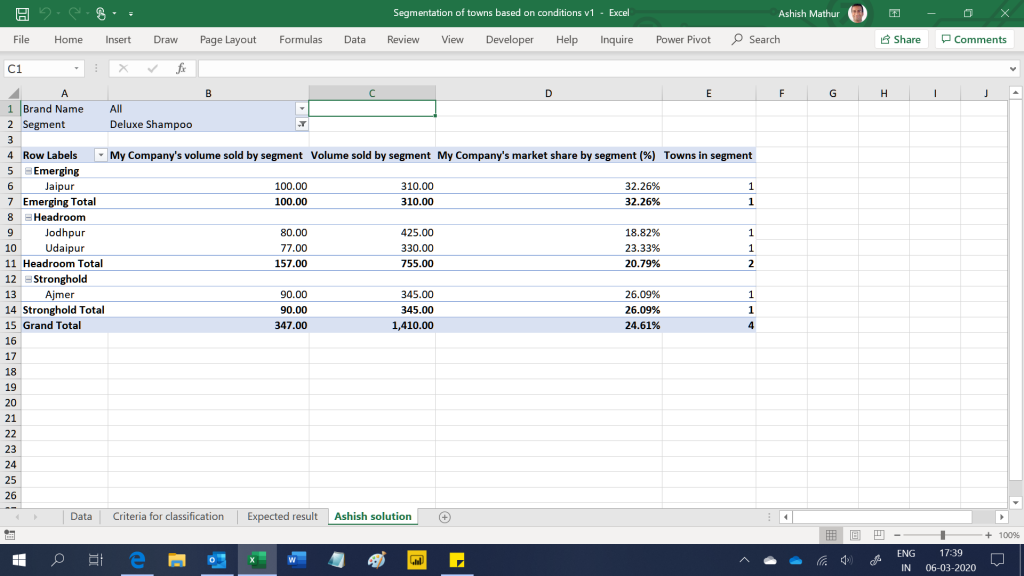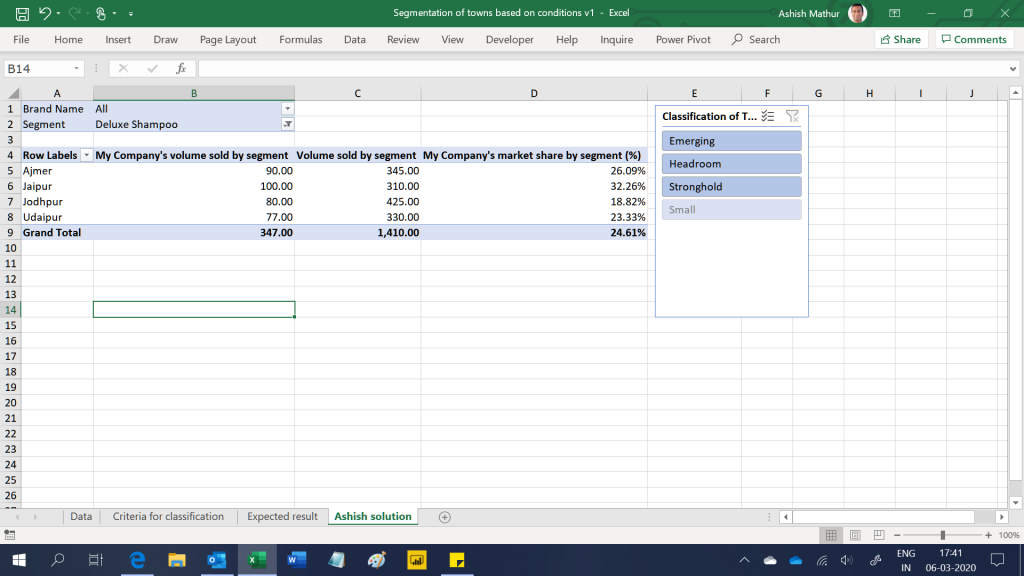Here's a simple dataset of Shampoo sales in the state of Rajasthan, India.For a chosen segment, one may want to segment the 4 towns based on the following conditions:Based on the two screenshots shared above, the desired result is shown in the screenshot below: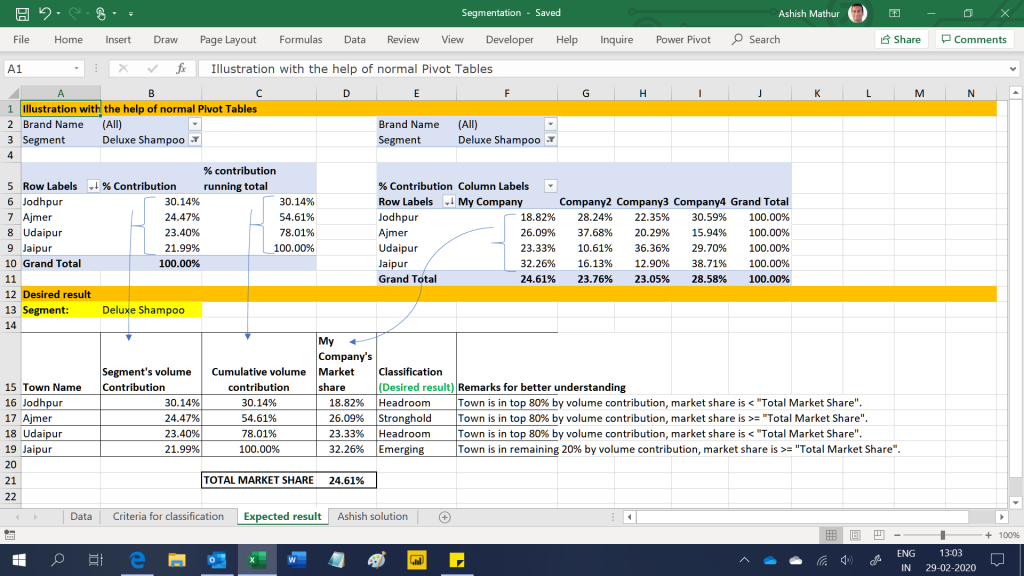The desired result is shown in range E16:E19 and the explanation of the classification is shown in range F16:F19.

The final result obtained by using the PowerPivot is shown in the screenshot below:Assume a simple dataset as shown in the image below (the input data is in columns A and B only.  The desired outcome is in columns C and D).The objective is to calculate the 7 days rolling sum and average (as shown in columns C and D) ignoring blank cells.  So in cell C8, the rolling sum is the summation of values from range B2:B8.  In cell C9, it is from B3:B9.  However, in cell C10, it will be from range B3:B9 (not from range B4:B10).  Likewise, in cell C11, the rolling sum will be from range B4:B11.  So the range to be considered for calculating the rolling sum has to roll back automatically until it picks up 7 numeric cells - the blanks have to be ignored.  The rolling average is a simple division - Rolling sum/7.

I have solved this question with Excel formulas here.  This time however, I am sharing a solution by using the DAX formula language available in the PowerPivot and PowerBI Desktop.  You may download my PowerBI Desktop file from here.  The same solution can also be obtained in MS Excel using the PowerPivot as well.

In the dataset below column A has the Employee Name, column B and C are the assignment start and end dates, Column D is the location and columns E to J are the Month-Year columns.  So each row represents data for an employee on a particular project.  The numbers in range E2:J8 represent how much that particular employee is aligned to the particular project i.e. a value of 1 means that the employee is dedicated solely to that project, 1.4 means that the employee will be spending extra hours on that project and 0.1 indicates that the employee will be working on multiple other projects.

The objective is to create another column (column K in the second screenshot) which will show the number of hours the employee will spend on the project.  The number of hours will be computed as number of working days in a month (treat Saturday and Sunday as weekends) * time allocation to that project (the numbers in range E2:J8) * 8.5 hours per day for an Offshore project and 8 hours per day for other projects.

The raw data sheet looks like thisThe expected result is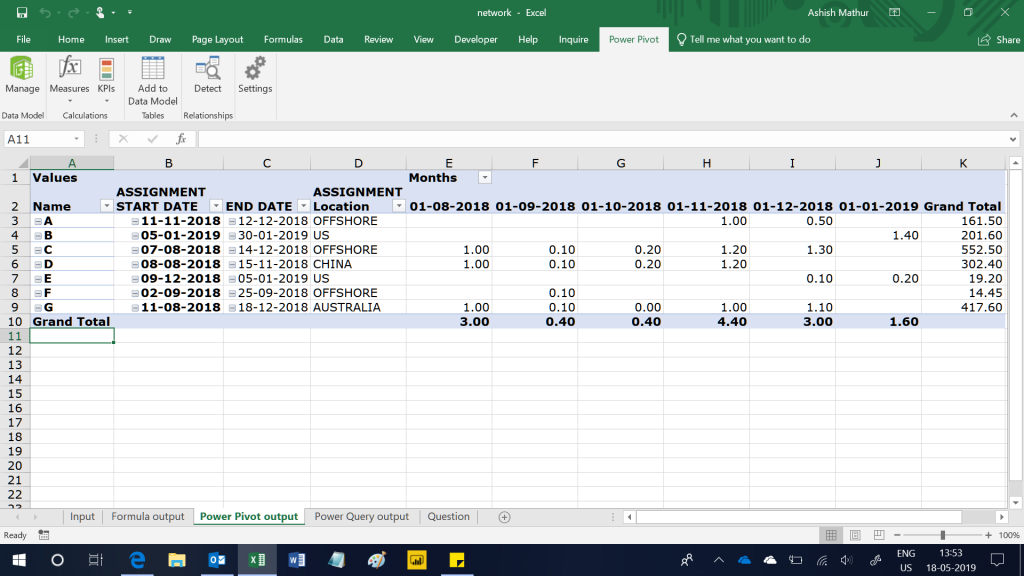The figure in cell K3 has been computed as:

• Number of working days between November 11, 2018 and November 30, 2018 are 15.  So 15 * 1 = 15
• Number of working days between December 1, 2018 and December 12, 2018 are 8.  So 8 * 0.5 = 4
• Total effective working days are 15 + 4 = 19
• Since it is an Offshore project, the hours per day would be 8.5.  Therefore total effective hours: 19 * 8.5 = 161.5

I have solved this problem using 3 methods:

1. Excel formulas - Refer worksheet named "Formula output"
2. Power Query and PowerPivot - Refer worksheet named "Power Pivot output"
3. Power Query only - Refer worksheet named "Power Query output"

Relative size factor (RSF) is a test to identify anomalies where the largest amount for subsets in a given key is outside the norm for those subsets. This test compares the top two amounts for each subset and calculates the RSF for each. In order to identify potential fraudulent activities in invoice payment data, one utilizes the largest and the second-largest amounts to calculate a ratio based on purchases that are grouped by vendors.  You may read more on this topic here.

Here is a 3 column dataset.  The first column is Vendor Number, the second is Invoice number and last is invoice amount.  There can be multiple invoices per vendor.  The objective is to determine the highest invoice value for a vendor and divide that by the second highest invoice value for that same vendor to get a ratio.  The same needs to be done for all vendors.  An interesting case in the dataset below is Vendor_No V4439 - there are 2 instances of highest value for this vendor i.e. 25,378.30 and another 2 instances of second highest value i.e. 24,068.25.  The RSF for this case will be 25,378.30/24,068.25.  If there is no instance of second highest value for a vendor, then the result should be 0.The expected result is:I have solved this question with the help of the PowerPivot.  You may download my solution workbook from here.

Here is a 2 column dataset - UserID in column A and Remarks in Column B.  This dataset basically tabulates the remarks/comments shared by different users.  Entries in the Remarks column are basically free flowing text entries which have the following inconsistencies/nuances:

1. Users reported multiple errors which are separated by comma, Alt+Enter (same line within the cell) and numbered bullets
2. Users committed spelling mistakes (see arrows in Table1)
3. A user ID may be repeated in column A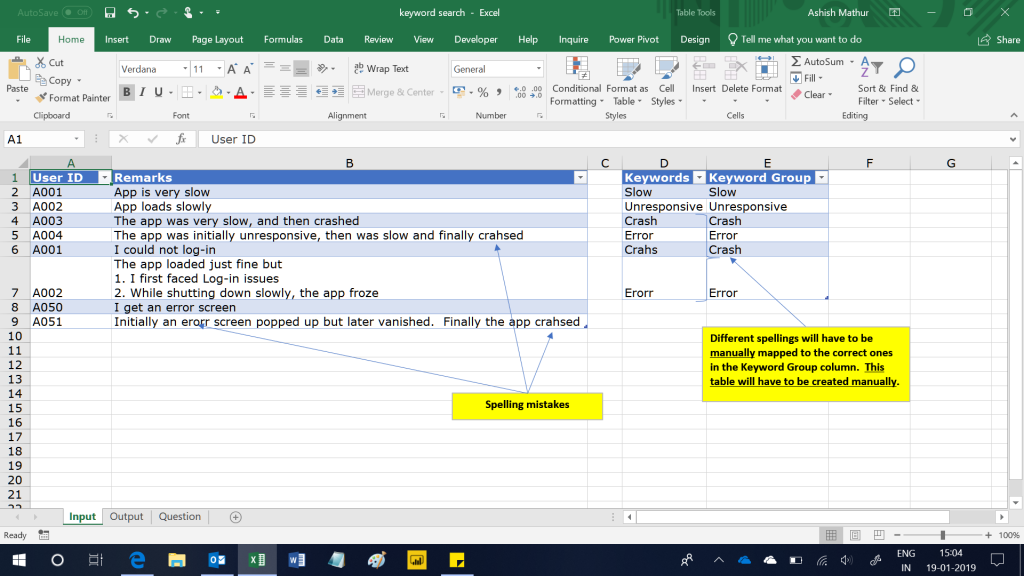Given this dataset, one may want to "hunt" for specific "keyword Groups" (column E above) in each user remark cell and get meaningful insights.  Some questions which one would like to have answers to are:

1. How may users reported each type of keyword Group - "How may users used the Unresponsive keyword?".  See Pivot Table1 below.
2. Which are the keyword Groups that each user reported - "Which are the different keyword groups reported by UserID A004?".  See Pivot Table2 below.
3. How many users reported each of the different keyword Groups - "How many users reported all 3 problems of Slow, unresponsiveness and crash".  See Pivot Table 3 below.
4. How may users who used this keyword group also used this keyword group - "How many users who reported Crash also reported Unresponsive?".  See Pivot Table 4 below.This was quite a formidable challenge to solve because of spelling mistakes and multiple keywords reported in each cell.  I have solved this problem with the help of Power Query and PowerPivot.  You may download my workbook from here.

Visualise a simple 6 column Table as shown below - Project Name and the finish date for each of the 5 stages that the projects go through.  Each project goes through 5 stages - Requirement (Req), Development (Dev), UAT, Implement and Warranty.The objective is to report on the status of each project at the end of each month based on which stage is/was completed in that month.  So, if a given project's requirements are completed in January and development completes some time in March, the one would expect the output of the report to show the project's status in January and February as "Req" and in March as "Dev" respectively.  February should also show "Req" because the next stage was completed only in March (although it may have started in January).  If multiple stages complete in one month, then the report should display only the most recently completed stage.  So, if Project A completed both Requirements and Development stages in January, the report should show only "Dev" as the stage completed in January.

For the data shared above, the expected result is: